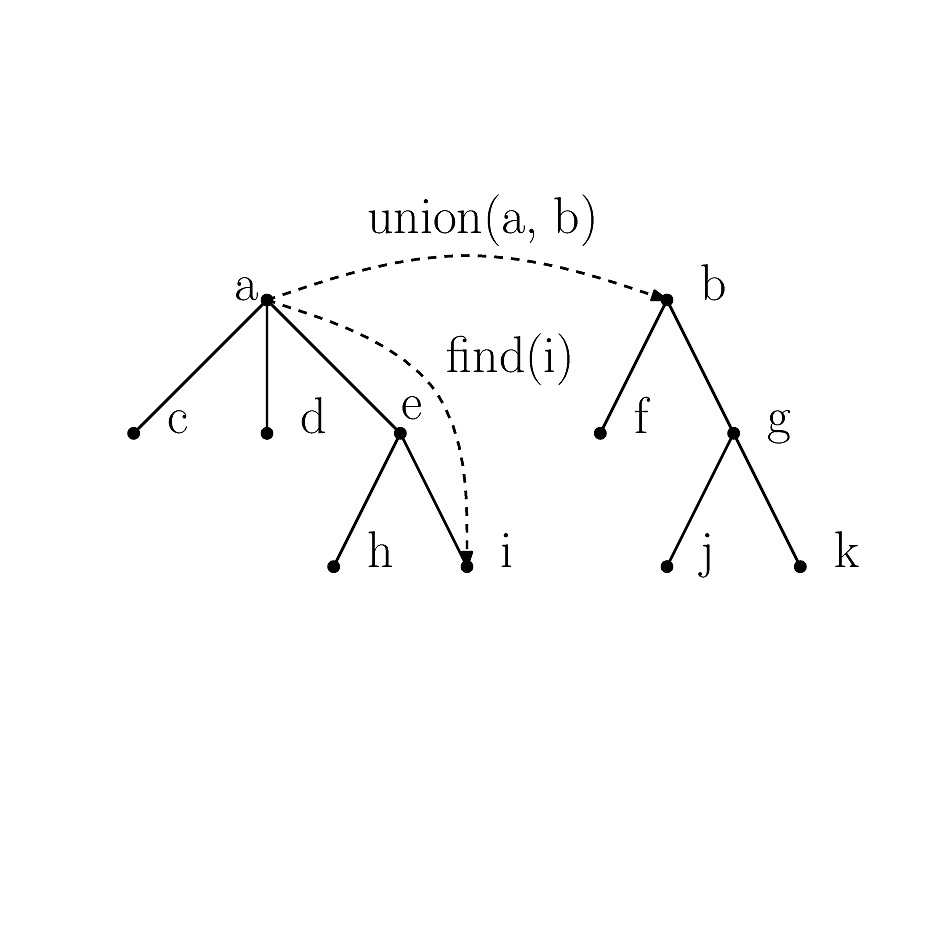Structural Bioinformatics Library Template C++ / Python API for developping structural bioinformatics applications.
User Manual# Union_find

Authors: F. Cazals and T. Dreyfus

# Introduction

The Union-Find (UF) algorithm maintains dynamically the connected components of an evolving graph, called independent sets in the sequel, upon addition of new edges, thanks to a Union-Find data structure (UF DS). The vertices of this graph may carry a piece of information called the attribute in the sequel.

Union-Find is based on the following three operations:

• make_set: creates a new independent set with one vertex.
• union_sets: merge the independent sets containing two given vertices.
• find_set: returns the independent set of a given vertex.

The key building blocks of the reference UF algorithm are as follows:

• each independent set is represented by a tree whose root is called the leader of the independent set. Each vertex points to its father, the father of the leader being NULL.
• the maintenance of the trees upon addition of new edges uses two strategies known as path-compression and union-by-rank.

Details can be found e.g. in the book Introduction to algorithms, by Cormen-Leiserson-Rivest-Stein  .

In addition to the aforementioned basic Union-Find operations, we also provide the following extensions:

• Algorithms: methods to process a list of edges, each of them possibly triggering a union_set operation on the vertices of the edge.
• Leaders: methods to maintain (and iterate on) the list of leaders.
• Independent set iterators: methods to maintain (and iterate on) the independent set containing a given vertex.
• Rewind (aka undo): methods to revert one-by-one a list of operations.

## Algorithms

We provide two algorithms to process a list of edges, each of them possibly triggering a union_set operation.

• given a list of edges, iterate over them and calls a union_set on the two vertices of each edge.
• given a predicate, iterate over all edges, and call a union_set on the two vertices of each edge for which the predicate evaluates to True.

An example scenario using these functionalities is given in the SBL::CADS::T_Union_find_specializes section.

A linked list of leaders is maintained. (Addition and removal from this list is done in constant time since the vertex data structure actually stores its location in that list.)

• retrieving the number of independent sets in constant time,
• iterating over the leaders without considering the other vertices.

Scenarios using this functionality are given in section SBL::CADS::T_Union_find_contains section and SBL::CADS::Union_find_default.

## Independent set iterators

Each vertex u is endowed with a linked list of all its children, i.e all the vertices having u as father. (As for leader, each vertex knows it location in that list.)

This extra information allows iterating recursively over all the descendants of a vertex u, i.e all the vertices v for which there is a path in the graph from u to v. In particular, starting from a leader allows visiting its independent set.

Combining this functionality with the iteration on leaders allows visiting the whole Union-Find data structure.

A scenario using this functionality is given in the SBL::CADS::T_Union_find_contains section.

## Rewind

Internally, the topology of the UF DS (omitting the maintenance of the ranks) is modified by the following operations:

• MAKE_SET(v) when creating the vertex v
• SET_FATHER(u, v) when setting the father of u to v, during the path compression or union_set operations.

Along a sequence of updates, we stack the aforementioned two operations. To rewind, it is sufficient to unstack these operations, and undo each of them.

A scenario using this functionality is given in section SBL::CADS::T_Union_find_contains.

# Implementation

The Union-Find data structure has been designed to handle any attribute type, without sacrificing the efficiency. There are three ways to represent an attribute in the Union-Find data structure:

• The vertex inherits from an attribute of type T : one can use SBL::CADS::T_Union_find_specializes < T > implementing a vertex data structure derived from the data type. Note that the previous limitation does not apply. However, inheritance cannot be used for pointer or primitive types (int, float, etc). See the SBL::CADS::T_Union_find_specializes section for an example.

# Examples

This example presents the SBL::CADS::Union_find_default class, with a graph encoded in a file. In particular, when the n vertices of the graph are represented by the n first not negative integers , the vertices of the graph can be used as the vertices of the UF DS.

The example reads a graph from a file, and dumps the leaders together with the size of their independent set.

This example presents the SBL::CADS::T_Union_find_contains class, using the Rewind functionality. It fills the Union-Find data structure with n vertices representing proteins, makes an arbitrary union of them, rewinds the Union-Find data structure to the last insertion, and re-makes a new arbitrary union of the vertices.

Since we need to attach an attribute to the Union-Find vertices, but we do not need to access a vertex from its associated data, we use the SBL::CADS::T_Union_find_contains class instantiated with the Protein const reference data type. (Note that instantiating directly with the data type will copy the data instead of copying the const reference to the data).